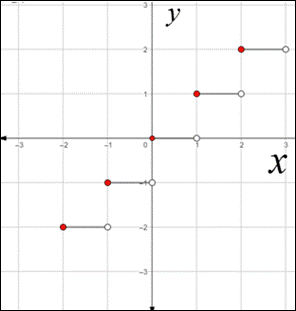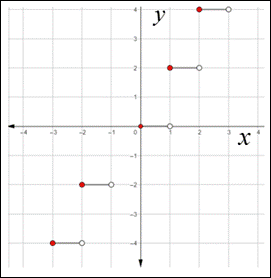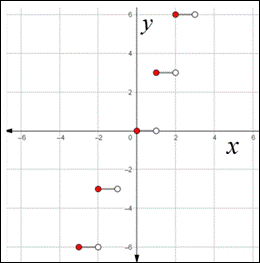# To sketch the graph of the function f ( x ) = 〚 x 〛 , g ( x ) = 〚 2 x 〛 and h ( x ) = 〚 3 x 〛 on separate graph and compare each graph.### Precalculus: Mathematics for Calcu...

6th Edition
Stewart + 5 others
Publisher: Cengage Learning
ISBN: 9780840068071### Precalculus: Mathematics for Calcu...

6th Edition
Stewart + 5 others
Publisher: Cengage Learning
ISBN: 9780840068071

#### Solutions

Chapter 2.2, Problem 86E
To determine

## To sketch the graph of the function f(x)=〚x〛 , g(x)=〚2x〛 and h(x)=〚3x〛 on separate graph and compare each graph.

Expert Solution

### Explanation of Solution

Given information:

The function whose graphs consist of horizontal line segment is called as step functions.

Concept used:

The greatest integer function is defined by,

x is the greatest integer less than or equal to x .

Graph:

To sketch the graph of the function f(x)=x , substitute different value of x and find the corresponding value of f(x)=x .

The table showing the values of f for some value of x is given below,

 x f(x)=〚x〛 ⋮ ⋮ −2≤x<−1 −2 −1≤x<0 −1 0≤x<1 0 1≤x<2 1 2≤x<3 2 ⋮ ⋮

The function is constant between consecutive integers, so the graph between integers is a horizontal line segment as shown below:To sketch the graph of the function g(x)=2x , substitute different value of x and find the corresponding value of g(x)=2x .

The table showing the values of f for some value of x is given below,

 x g(x)=〚2x〛 ⋮ ⋮ −2≤x<−1 −4 −1≤x<0 −2 0≤x<1 0 1≤x<2 2 2≤x<3 4 ⋮ ⋮

The function is constant between consecutive integers, so the graph between integers is a horizontal line segment as shown below:To sketch the graph of the function h(x)=3x , substitute different value of x and find the corresponding value of h(x)=3x .

The table showing the values of f for some value of x is given below,

 x h(x)=〚3x〛 ⋮ ⋮ −2≤x<−1 −6 −1≤x<0 −3 0≤x<1 0 1≤x<2 3 2≤x<3 6 ⋮ ⋮

The function is constant between consecutive integers, so the graph between integers is a horizontal line segment as shown below:Interpretation:

On comparing each of the above three graphs, it can be observed that each of the horizontal line segment shifted by one unit for every one unit increase in integer within the bracket.

For the function, k(x)=nx ,

The graph of k(x)=nx will look like stairs and the vertical distance between each horizontal line will be equal to n .

### Have a homework question?

Subscribe to bartleby learn! Ask subject matter experts 30 homework questions each month. Plus, you’ll have access to millions of step-by-step textbook answers!